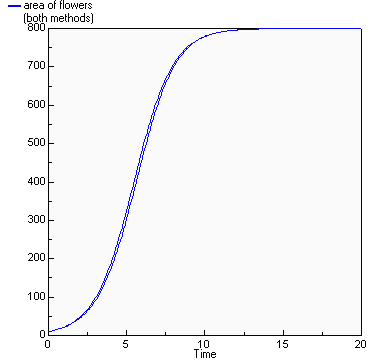# Logistic growth

## Area covered in "flowers" compared with logistic growth model

ModelId:
flowers2
SimileVersion:
3.1+

This models the spatial spread of vegetation over an area. Here, the vegetation is taken to be �flowers�. This is not a spatial model, in the usual sense where space is divided up into discrete units. Rather, the area covered by the flowers is represented by a single state variable, with an inflow representing increase in area (spread), and an outflow representing decrease in area (death of flowers).

Equations:

Compartments:

area of flowers
Initial value = 10
Rate of change = + growth + decay
Comments: hectares (OK, Ford says acres, but we are in the 21st century now).

Flows:
decay = area_of_flowers*decay_rate

growth = area_of_flowers*actual_growth_rate

Variables:
A of t = Azero*exp(r*time(1))/(1+Azero*(exp(r*time(1))-1)/K)

actual growth rate = intrinsic_growth_rate*growth_rate_multiplier

area of flowers (both methods) = [area_of_flowers,A_of_t]

fraction occupied = area_of_flowers/suitable_area

growth rate multiplier = graph(fraction_occupied)

intrinsic growth rate = 1

Parameters:
Azero = 10
Comments: Initial area of flowers (hectare)

decay rate = 0.2

K = 800
Comments: "Maximum value that the flowered area would reach over time"

r = intrinsic_growth_rate-decay_rate

suitable area = 1000
Comments: hectares (see 'area of flowers'...)

Results:References:

Ford, A (1999) Modeling the Environment.